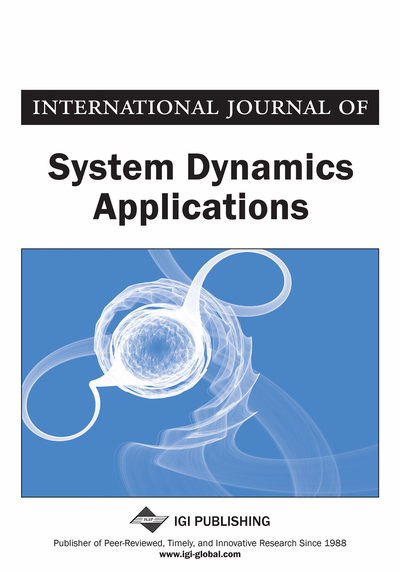# Fuzzy Modelling and Chaos Control in the Photogravitational Magnetic Binary Problem With Potential From a Belt

Mohd Arif (Zakir Husain Delhi College, Delhi University, India)
DOI: 10.4018/IJSDA.2020070102

## Abstract

Control of chaotic systems has led to many fruitful results, such as the famous OGY and feedback control. Of course, controlling chaos is not limited to the approaches above and is not specifically reviewed one by one here. The representation of chaotic systems using fuzzy models has a unified approach. The fuzzy logic controllers had been proposed for a long time and were successfully applied to control the chaos in many systems. The main purpose of this study is to presents the new fuzzy model for the photogravitational magnetic-binary problem (PMBP) where the bigger primary is a source of radiation and the smaller primary is an oblate body; and they are encompassed by a homogeneous circular cluster of material points centred at the mass centre of the system (belt). It was shown that using a new fuzzy controller, it is possible to control of chaotic behaviour of the photogravitational magnetic-binary problem (PMBP). The simulation results have demonstrated that the proposed method can satisfy the control object and enhanced stability.
Article Preview
Top

## 1. Introduction

A chaotic system in nature is a complex system that exhibits a complicated, random and disorganized behaviour. The chaotic systems are highly sensitive to small changes in their initial conditions and parameters variation. Chaos synchronization, which refers to a process wherein two (or many) chaotic systems (either equivalent or non-equivalent) adjust a given property of their motion to a common behaviour due to a coupling or to a forcing (periodical or noisy) Boccaletti et al. (2002). Chaos control and synchronization both are important research fields levelling to affect dynamics of chaotic systems in order to apply them for different kinds of applications that can be examined within many different scientific research (Ahmad et al., 2014; Andrievskii & Fradkov, 2004). Recently different kinds of control methods and techniques that have been proposed for carrying out chaos control and synchronization of chaotic dynamical systems. Some control methods such as feedback linearization (Shi & Wang, 2013), sliding-mode approach (Jalel et al., 2018; Crassidis & Markley, 1996; Vaidyanathan & Azar, 2015a, 2015b), lyapunov-based control technique approach, (Fragopoulos & Innocenti, 2004), LMI based nonlinear technique (Show et al.,2003; Shih & Zheng-Ming, 2011), Adaptive Neuro-Fuzzy Sliding Mode Controller (Sana Bouzaida &Anis Sakly, 2018), Hierarchal Fuzzy Logic Controller (Rabie & Ahmed, 2017) and A Neuro-Fuzzy Approach (Ashwani & Pravin, 2017).

There has been a considerable number of studies on fuzzy control where fuzzy implications are used to express control rules. The Takagi-Sugeno (T-S) fuzzy system is one of the most popular fuzzy systems in model-based fuzzy control (Kazuo & Wang, 2001; Takagi & Sugeno, 1985). In the current scenario, T-S fuzzy model is widely applied to many fields because of its simple structure with local and global dynamics. In the last few years much research on the fuzzy model-based design has been carried out on the basis of the T-S fuzzy model (Sun & Wang, 2006; Vascak, 2008). They are characterized by fuzzy logic control rules of IF-THEN type where the consequents are usually local affine or linear models. Their advantages come from the fact that the T-S fuzzy models can express highly non-linear functional relations with a relatively small number of rules. In the T–S fuzzy model number of the linear subsystem is decided by how many minimum nonlinear terms should be linearized in original system. If there arelinear subsystems then according tofuzzy rules there will beequations in the system, where N is the number of minimum nonlinear terms, andis the order of the system. For large, the number of linear subsystems becomes very complicated in the T–S fuzzy system.

Stormer (1907) has studied the motion of a charged particle moving in the field of a magnetic dipole as a two body problem. This problem in general is quite complicated and is non- integrable. (Mavraganis, 1978) has studied the motion of a charged particle moving in the field of two rotating magnetic dipoles instead of one dipole and he defined the problem as: Two bodies (primaries), with magnetic fields, move under the influence of gravitational force and a charged particle moves in the vicinity of these bodies. The question of the magnetic -binary problem is to describe the motion of this problem.

## Complete Article List

Search this Journal:
Reset
Open Access Articles
Volume 10: 4 Issues (2021): 2 Released, 2 Forthcoming
Volume 9: 4 Issues (2020): 3 Released, 1 Forthcoming
Volume 8: 4 Issues (2019)
Volume 7: 4 Issues (2018)
Volume 6: 4 Issues (2017)
Volume 5: 4 Issues (2016)
Volume 4: 4 Issues (2015)
Volume 3: 4 Issues (2014)
Volume 2: 4 Issues (2013)
Volume 1: 4 Issues (2012)
View Complete Journal Contents Listing